# Preface

This tutorial was made solely for the purpose of education and it was designed for students taking Applied Math 0330. It is primarily for students who have very little experience or have never used Mathematica and programming before and would like to learn more of the basics for this computer algebra system. As a friendly reminder, don't forget to clear variables in use and/or the kernel. The Mathematica commands in this tutorial are all written in bold black font, while Mathematica output is in normal font.

Finally, you can copy and paste all commands into your Mathematica notebook, change the parameters, and run them because the tutorial is under the terms of the GNU General Public License (GPL). You, as the user, are free to use the scripts for your needs to learn the Mathematica program, and have the right to distribute this tutorial and refer to this tutorial as long as this tutorial is accredited appropriately. The tutorial accompanies the textbook Applied Differential Equations. The Primary Course by Vladimir Dobrushkin, CRC Press, 2015; http://www.crcpress.com/product/isbn/9781439851043

We need the following result that follows from the linearization procedure.

Theorem: Suppose y* is an equilibrium point (that is, $$f\left( y^{\ast} \right) =0$$ ) of the differential equation $${\text d}y / {\text d}t = f\left( y \right) ,$$ where f is a continuously differentiable function. Then,

• if $$f\left( y^{\ast} \right) < 0 ,$$ then y* is a sink;
• if $$f\left( y^{\ast} \right) > 0,$$ then y* is a source;
• if $$f\left( y^{\ast} \right) =0 ,$$ then we need additional information to determine the type of y*.

# Bifurcation

Many of the equations that we have examined have a parameter, which means that we actually have a family of differential equations. For example, the logistic equation
$\frac{{\text d} P}{{\text d} t} = r \, P \left( 1 - \frac{P}{N} \right)$
has two parameters, r and N. In this section we will investigate how the solutions of a differential equation vary as we change the value of a parameter or parameters.

Consider an autonomous differential equation depending on a parameter k: $${\text d}y /{\text d}t = f(y;k) .$$ We say that a bifurcation occurs at the parameter value k = k0 if there is a change in the qualitative nature of the families of solutions when the parameter varies in a neighborhood of k0.

A bifurcation diagram for a parameterized family of autonomous differential equations depending on a parameter k, $${\text d}y /{\text d}t = f(y;k) ,$$ is a diagram in the ky-plane that summarizes the change in qualitative behavior of the equation as the parameter k is varied. The word bifurcate means “to divide into two parts or branches.” Hence, a bifurcation diagram shows us at what parameter values additional solutions emerge (or disappear). The bifurcation diagram is constructed by plotting the parameter value k against all corresponding equilibrium values $$y^{\ast} .$$ Typically, k is plotted on the horizontal axis and critical points y* on the vertical axis. A "curve" of sinks is indicated by a solid line and a curve of sources is indicated by a dashed line. The method of constructing bifurcation diagrams should become evident as we study the following examples.

We need to make an observation that bifurcation occurs at the point $$\left( k_{0} , y^{\ast} \right)$$ only when

$f\left( k_0 , y^{\ast} \right) =0 , \qquad \mbox{and} \qquad \left. \frac{\partial f(y; k)}{\partial y} \right\vert_{y= y^{\ast}} = 0 .$
A bifurcation does not happen:
• if y* is a sink with $$f\left( y^{\ast} \right) < 0 ,$$ then for all k sufficiently close to k0 the critical point y* remains a sink because the inequality $$f\left( y^{\ast} \right) < 0$$ holds in some neighborhood of the equilibrium point;
• if y* is a source with $$f\left( y^{\ast} \right) > 0,$$ then for all k sufficiently close to k0 the critical point y* remains a source because the inequality $$f\left( y^{\ast} \right) > 0$$ holds in some neighborhood of the equilibrium point.

Example (Logistic Model with Harvesting). We model logistic growth in a trout pond with the equation

$\frac{{\text d} P}{{\text d} t} = 0.01 \, P \left( 1 - \frac{P}{400} \right) .$
There are two equilibrium solutions P = 400 (a sink) and P = 0 (a source).
StreamPlot[{1, y*(1 - y/400)/100}, {x, 0, 250}, {y, 300, 500}, Frame -> True, Axes -> True]
StreamPlot[{1, y*(1 - y/400)/100}, {x, 0, 250}, {y, -50, 50}, Frame -> True, Axes -> True]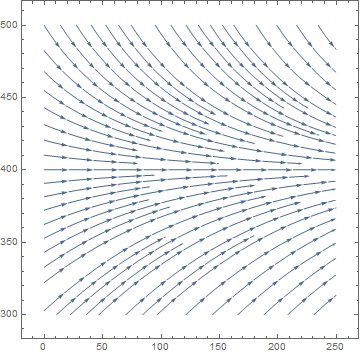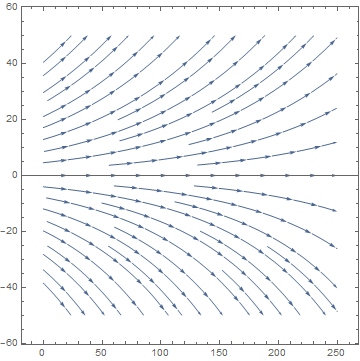If we allowed fishing in our pond at a rate of H = 3/4 tons of fish per year, then the equation became
$\frac{{\text d} P}{{\text d} t} = 0.01 \, P \left( 1 - \frac{P}{400} \right) -H , \qquad H=\frac{3}{4} .$
There are two equilibrium solutions for this equation, P = 300 (a sink) and P = 100 (a source). If the population of the pond falls below 100, then the fish will die out unless the pond is restocked or fishing is banned.
StreamPlot[{1, y*(1 - y/400)/100 - 3/4}, {x, 0, 250}, {y, 0, 410}, Frame -> True, Axes -> True]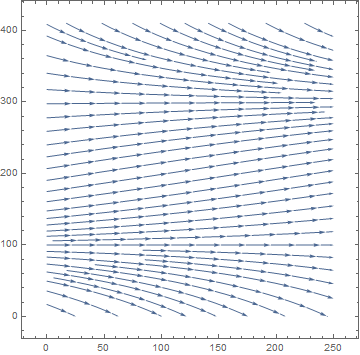Now let us see what happens when we allow more fishing in our pond, say H = 10. Our differential equation now becomes
$\frac{{\text d} P}{{\text d} t} = 0.01 \, P \left( 1 - \frac{P}{400} \right) -10.$
To find equilibrium solutions, we have to solve the quadratic equation
$0.01 \, P \left( 1 - \frac{P}{400} \right) -10 =0 .$
Thus,
$P = 200 \pm 600\,{\bf j} ,$
which means that quadratic equation has no real solutions and that we have no equilibrium solutions. Furthermore, $${\text d}P /{\text d} t < 0$$ for all values of P. This means that no matter how many fish are in the pond initially, the trout population will eventually die out due to over fishing.

To find a critical value of H, we solve the quadratic equation

$0.01 \, P \left( 1 - \frac{P}{400} \right) - H =0$
to obtain two critical points
$P_{1,2} = 200 \left( 1 \pm \sqrt{1-H} \right) .$
By plotting the above function, we obtain the bifurcation diagram.
a = Plot[1 - Sqrt[1 - H], {H, -1, 1}, PlotStyle -> {Dashed, Thick, Blue}];
b = Plot[1 + Sqrt[1 - H], {H, -1, 1}, PlotStyle -> {Thick, Blue}];
Show[a, b, PlotRange -> {{-1, 1}, {-0.5, 2.5}}]
If H < 1, we have two nodes $$P_1 = 200 \left( 1 - \sqrt{1-H} \right)$$ (a source, unstable) and $$P_2 = 200 \left( 1 + \sqrt{1-H} \right)$$ (sink, asymptotically stable). If H = 1, both critical points merge into semistable equilibrium solution $$P = 200 .$$
StreamPlot[{1, P*(1 - P/400)/100 - 1}, {t, 0, 250}, {P, 150, 250}, Frame -> True, Axes -> True]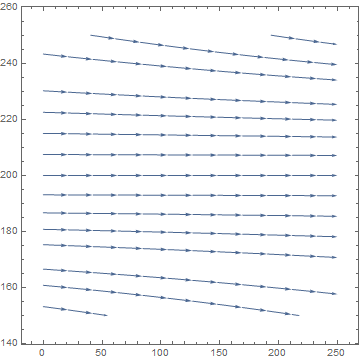When H exceeds 1, all trajectories decline and the population dies out independently on the initial conditions.
StreamPlot[{1, y*(1 - y/400)/100 - 2}, {x, 0, 250}, {y, 0, 400}, Frame -> True, Axes -> True]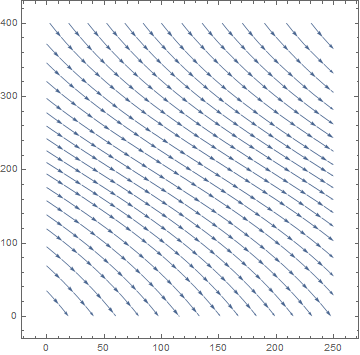A small change in H can have a dramatic effect on how the solutions of the differential equation behave. Changing the value of H from 1 to 1.1 will doom the population of fish to extinction no matter what the initial population is. As we increase the value of H, the number of equilibrium solutions changes from two to one and then to none. This change occurs exactly at H = 1. We say that a bifurcation occurs at H = 1 for the given logistic equation.

Example: Let us consider the autonomous equation

$\frac{{\text d} y}{{\text d} x} = y^2 -6\,y + k$
as a family of differential equations indexed by the parameter k. If we let $$f_{k}(y) = f (y;k) = y^2 -6\,y + k ,$$ then
$\frac{{\text d} y}{{\text d} x} = f_{k} (y) = f(y;k)$
is a called one-parameter family of differential equations. For each value of k, we obtain an autonomous differential equation, and for each value of $$k \le 9,$$ we have different pair of critical points:
$y^{\ast} = 3- \sqrt{9-k} \quad \mbox{(sink)}, \qquad y^{\ast} = 3+ \sqrt{9-k} \quad \mbox{(source)}.$
Its bifurcation diagram consists of two branches of roots: $$3 \pm \sqrt{9-k} .$$

For k = 0, the differential equation becomes

$\frac{{\text d} y}{{\text d} x} = f_0 (y) = y^2 -6\,y = y \left( y-6 \right) .$
There is a sink at y = 0, and a source at y = 6.
StreamPlot[{1, y*(y - 6)}, {x, 0, 5}, {y, 0, 8}, Frame -> True, Axes -> True]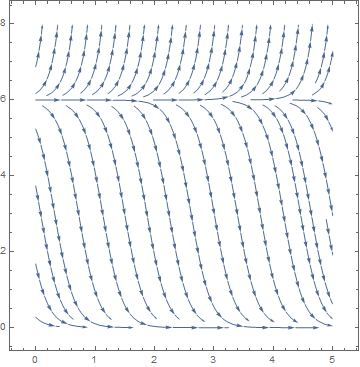For k = 9, the differential equation
$\frac{{\text d} y}{{\text d} x} = f_{9} (y) = f(y; 9) = (y-3)^2$
has exactly one equilibrium solution, a semistable node at y = 3.
StreamPlot[{1, (y - 3)^2}, {x, 0, 20}, {y, -1, 6}, Frame -> True, Axes -> True]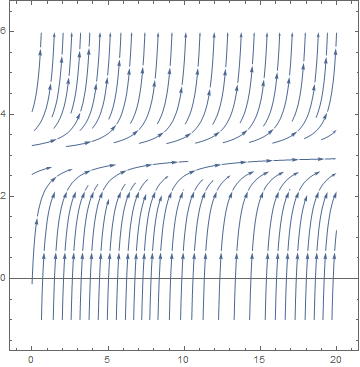When k > 9, then the differential equation $$y' = f_{k} (y)$$ has no equilibrium solutions
StreamPlot[{1, (y - 3)^2 +1}, {x, 0, 20}, {y, -1, 6}, Frame -> True, Axes -> True]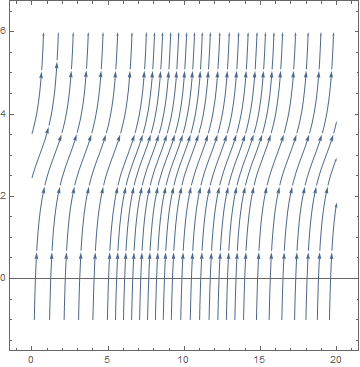Example: Let us find the bifurcation values of the one-parameter family

$\frac{{\text d} y}{{\text d} x} = y\left( y-3\right)^2 + k .$
If $$g_{k} (y) = g(y;k) = y\left( y-3\right)^2 + k ,$$ then $$g'_{k} (y) = \left( y-3\right)^2 + y \left( y-3\right) = 2\,y^2 -9y +9 .$$ The roots of $$g'_{k} (y) = 0$$ are y =3 and y = 3/2. In order for k to be a bifurcation value, we must have $$g_{k} (3) = k = 0$$ or
$g_{k} (3/2) = \frac{27}{8} + k =0.$
Therefore, the given equation has two bifurcation points k = -8/27 and k = 0. Upon solving the cube equation $$y\left( y-3\right)^2 + k =0 ,$$ we obtain the real critical point
$y^{\ast} = 2+ \frac{2^{1/3}}{\left( \sqrt{4k+ k^2} - 2 -k \right)^{1/3}} + \frac{\left( \sqrt{4k+ k^2} - 2 -k \right)^{1/3}}{2^{1/3}} .$
By plotting the above function, we get the bifurcation diagram for this one-parameter family: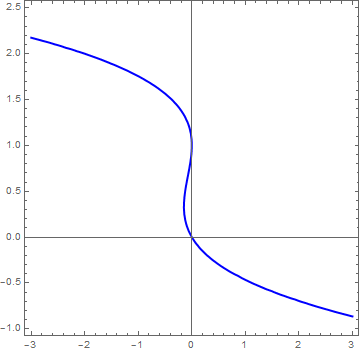Bifurcation diagram for $$y' = y\left( y-3\right)^2 + k.$$

A saddle-node bifurcation is a local bifurcation in which two (or more) critical points (or equilibria) of a differential equation (or a dynamic system) collide and annihilate each other. Saddle-node bifurcations may be associated with hysteresis and catastrophes.

Consider the slope function $$f(x, \alpha ) ,$$ where α is a control parameter. In this example, we use α instead of k because we need clearly distinguish a parameter (Greek letter α) from the variable (Latin letter y); we will return to k after rescaling. Then the solutions of the autonomous differential equation $$x' = f(x, \alpha )$$ and critical points (if any) will also depend on this parameter α. If we demand $$f(x, \alpha ) =0$$ and $$\partial_x f(x, \alpha ) =0,$$ we get two equations in two unknowns, and in general there will be a zero-dimensional solution set consisting of points $$\left( x_c , \alpha_c \right) .$$ Let’s expand $$f(x, \alpha )$$ in the vicinity of such a point $$\left( x_c , \alpha_c \right) :$$

\begin{align*} f(x, \alpha ) &= f \left( x_c , \alpha_c \right) + \left. \frac{\partial f}{\partial x} \right\vert_{\left( x_c , \alpha_c \right)} \left( x- x_c \right) + \left. \frac{\partial f}{\partial \alpha} \right\vert_{\left( x_c , \alpha_c \right)} \left( \alpha - \alpha_c \right) \\ &\quad + \frac{1}{2}\, \left. \frac{\partial^2 f}{\partial x^2} \right\vert_{\left( x_c , \alpha_c \right)} \left( x- x_c \right)^2 + \left. \frac{\partial^2 f}{\partial x \partial \alpha} \right\vert_{\left( x_c , \alpha_c \right)} \left( x- x_c \right) \left( \alpha - \alpha_c \right) + \frac{1}{2}\, \left. \frac{\partial^2 f}{\partial \alpha^2} \right\vert_{\left( x_c , \alpha_c \right)} \left( \alpha - \alpha_c \right)^2 + \cdots \\ &= A \left( \alpha - \alpha_c \right) + B \left( x- x_c \right)^2 + \cdots , \end{align*}
where we keep terms of lowest order in the deviations δx and δα. If we now rescale $$u \equiv \sqrt{B/A} \left( x- x_c \right) , \quad k \equiv \left( \alpha - \alpha_c \right) ,$$ and $$\tau = Ax ,$$ we obtain, neglecting the higher order terms, the ‘normal form’ or generic model of the saddle-node bifurcation:
$\frac{{\text d} u}{{\text d} \tau} = k + u^2 .$
a = Plot[x^2 - 2, {x, -3, 3}, Frame -> False, Axes -> False, PlotStyle -> {Thick, Brown}]
b = Graphics[{Blue, Thick, Arrow[{{Sqrt - 0.05, 0}, {-0, 0}}], Arrow[{{0, 0}, {-Sqrt + 0.05, 0}}]}]
c = Graphics[Line[{{0, -2.02}, {0, 4}}]]
t1 = Graphics[Text[Style["u", FontSize -> 14, Black], {2.8, -0.3}]]
t2 = Graphics[Text[Style["f", FontSize -> 14, Black], {0.2, 2.9}]]
t3 = Graphics[Text[Style["r<0", FontSize -> 14, Black], {0.0, -2.3}]]
b1 = Graphics[{Red, Thick, Arrow[{{Sqrt + 0.1, 0}, {2, 0}}], Arrow[{{2, 0}, {3, 0}}]}]
b2 = Graphics[{Red, Thick, Arrow[{{-2, 0}, {-Sqrt - 0.1, 0}}], Arrow[{{-3, 0}, {-2, 0}}]}]
st = Graphics[{PointSize[Large], Point[{Sqrt, 0}]}]
circle = Graphics[Circle[{-Sqrt, 0}, 0.05], AspectRatio -> 1]
Show[a, b, c, t1, t2, t3, b1, b2, circle, st, AspectRatio -> 1, PlotRange -> {{-3, 3}, {-2.1, 3}}]
a = Plot[x^2 , {x, -3, 3}, Frame -> False, Axes -> False, PlotStyle -> {Thick, Brown}]
c = Graphics[Line[{{0, -0.02}, {0, 4}}]]
t1 = Graphics[Text[Style["u", FontSize -> 14, Black], {2.8, -0.3}]]
t2 = Graphics[Text[Style["f", FontSize -> 14, Black], {0.2, 2.9}]]
t3 = Graphics[Text[Style["r=0", FontSize -> 14, Black], {0.0, -0.3}]]
b1 = Graphics[{Red, Thick, Arrow[{{0.01, 0}, {2, 0}}], Arrow[{{2, 0}, {3, 0}}]}]
b2 = Graphics[{Red, Thick, Arrow[{{-2, 0}, {-0.1, 0}}], Arrow[{{-3, 0}, {-2, 0}}]}]
circle = Graphics[Circle[{0, 0}, 0.05], AspectRatio -> 1]
Show[a, c, t1, t2, t3, b1, b2, circle, AspectRatio -> 1, PlotRange -> {{-3, 3}, {-2.1, 3}}]
a = Plot[x^2 +0.5, {x, -3, 3}, Frame -> False, Axes -> False, PlotStyle -> {Thick, Brown}]
c = Graphics[Line[{{0, -0.02}, {0, 4}}]]
t1 = Graphics[Text[Style["u", FontSize -> 14, Black], {2.8, -0.3}]]
t2 = Graphics[Text[Style["f", FontSize -> 14, Black], {0.2, 2.9}]]
t3 = Graphics[Text[Style["r=0", FontSize -> 14, Black], {0.0, -0.3}]]
b1 = Graphics[{Red, Thick, Arrow[{{0.02, 0}, {2, 0}}], Arrow[{{2, 0}, {3, 0}}]}]
b2 = Graphics[{Red, Thick, Arrow[{{-2, 0}, {-0.02, 0}}], Arrow[{{-3, 0}, {-2, 0}}]}]
Show[a, c, t1, t2, t3, b1, b2, AspectRatio -> 1, PlotRange -> {{-3, 3}, {-2.1, 3}}]

The right-hand side of this differential equation is the function $$f(u;k) = k+ u^2$$ that depends continuously on the parameter k. If we graph this function as a function of u for different values of k to determine equilibria, we see that for k < 0, there are two equilibria, which disappear when k becomes positive.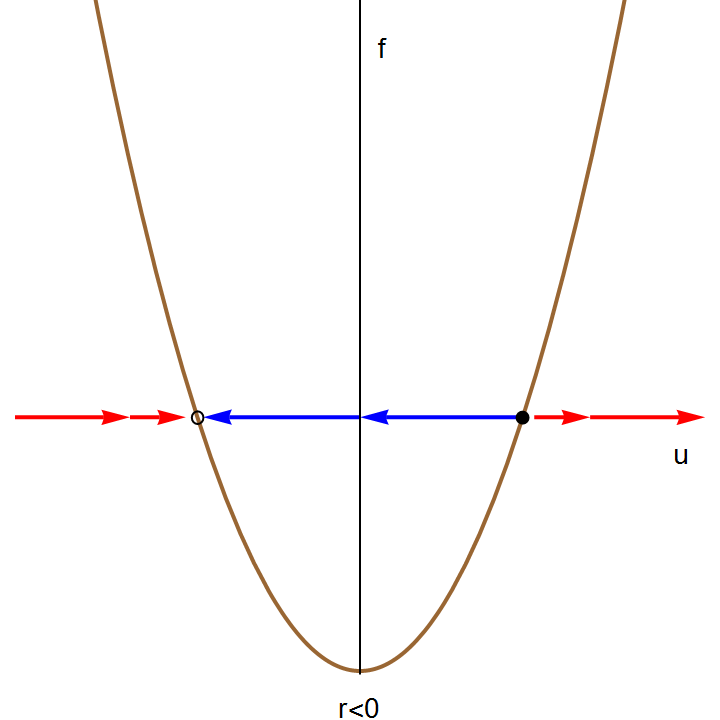Flow diagrams for the saddle-node bifurcation $$\dot{y} = r + y^2 ,$$ (top) and the transcritical bifurcation $$\dot{y} = ry - y^2$$ (bottom).

We can also graph the equilibria as functions of k. This figure is another example of a bifurcation diagram. For k < 0, there are two fixed points -- one stable ($$u^{\ast} = - \sqrt{-k}$$ ) and one unstable ($$u^{\ast} = + \sqrt{-k}$$ ). At k = 0, these two nodes coalesce and annihilate each other. (The point u* = 0 is half-stable precisely at k = 0.) For k > 0, there are no longer any fixed points in the vicinity of u = 0. In the left panel of Figure, we show the flow in the extended (k,u) plane. The unstable and stable nodes annihilate at k = 0.

a = Plot[-Sqrt[-x], {x, -2, 0}, PlotStyle -> {Thick, Black}]
b = Plot[Sqrt[-x], {x, -2, 0}, PlotStyle -> {Dashed, Thick, Blue}]
Show[a, b, PlotRange -> {{-2, 0.1}, {-1.4, 1.4}}]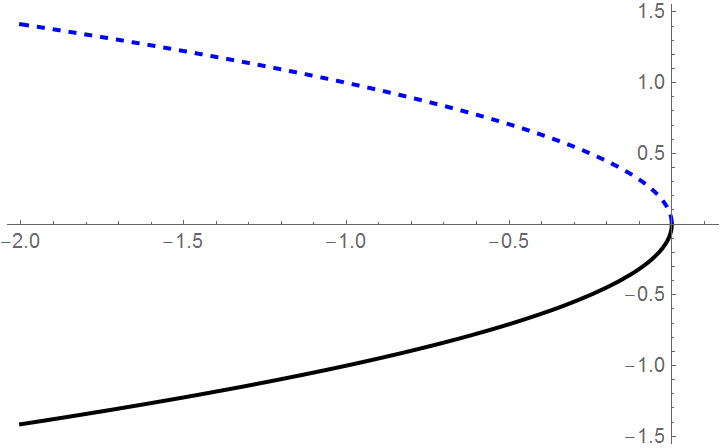Bifurcation diagram for the saddle-node bifurcation: As k crosses 0, the two equilibria disappear. The locally stable solution is indicated by the solid line, the unstable one by the dashed line.

It is possible to calculate the time it takes a solution starting at $$-\infty$$ at τ=0 to reach $$\infty$$ at τ = T. We can find T by solving the differential equation $$\dot{u} = k + u^2$$ with the conditions $$u(0) = -\infty$$ and $$u(T) = \infty .$$ We separate variables and compute the following definite integrals:

$\int_{-\infty}^{\infty} \frac{{\text d} u}{k+ u^2} = \int_0^T {\text d} \tau .$
The integral on the right-hand side is equal to T. The integral on the left-hand side needs more work. We will use the substitution $$x= u/\sqrt{k}$$ and hence $$\sqrt{k}\,{\text d}x = {\text d}u .$$ Upon factoring k in the denominator, we get
$\int \frac{{\text d} u}{k+ u^2} = \int \frac{\sqrt{k}\,{\text d} x}{k \left( 1+ x^2 \right)} = \frac{1}{\sqrt{k}}\, \arctan x +C = \frac{1}{\sqrt{k}}\, \arctan \frac{u}{\sqrt{k}} +C .$
Therefore,
$T= \int_{-\infty}^{\infty} \frac{{\text d} u}{k+ u^2} = \left. \frac{1}{\sqrt{k}}\, \arctan \frac{u}{\sqrt{k}} \right\vert_{u=-\infty}^{u=\infty} = \frac{1}{\sqrt{k}} \left[ \frac{\pi}{2} - \left( -\frac{\pi}{2} \right) \right] = \frac{\pi}{\sqrt{k}} .$
We thus find that the time it takes the solution to go from $$-\infty$$ at τ=0 to reach $$\infty$$ at τ = T is $$\pi / \sqrt{k} .$$ This shows that as k approaches 0 from the right, it takes the solution longer and longer. The slowdown occurs when the solution passes through u = 0.

## II. Transcritical bifurcation

A transcritical bifurcation is a particular kind of local bifurcation when stability of critical points changes as the parameter is varied. In other words, the unstable fixed point becomes stable and vice versa. A critical point under a transcritical bifurcation is never destroyed, it just interchanges its stability with another critical point.

Another situation which arises frequently is the transcritical bifurcation. Consider the equation $$\dot{y} = f(y)$$ in the vicinity of a fixed point y*.

$\frac{{\text d} y}{{\text d} t} = f' \left( y^{\ast} \right) \left( y - y^{\ast} \right) + \frac{1}{2}\, f'' \left( y^{\ast} \right) \left( y - y^{\ast} \right)^2 + \cdots .$
We rescale $$u \equiv \beta \left( y - y^{\ast} \right)$$ with $$\beta = - \frac{1}{2}\, f'' \left( y^{\ast} \right)$$ and define $$k = f' \left( y^{\ast} \right)$$ as the control parameter, to obtain, to order u2
$\frac{{\text d} u}{{\text d} t} = k\, u - u^2 .$
Note that the sign of the u2 term can be reversed relative to the others by sending $$u \,\mapsto -u$$ and $$k \,\mapsto -k .$$ The right-hand side of this differential equation is the function $$f(u;k) = k\, u - u^2$$ that depends continuously on the parameter k. If we graph this function as a function of u for different values of k to determine equilibria, we see that there are two equilibria. These equilibria change stability as k changes from a negative to a positive value.

Example. Consider a crude model of a laser threshold. Let n be the number of photons in the laser cavity, and N the number of excited atoms in the cavity. The dynamics of the laser are approximated by the equations

$\begin{split} \dot{n} &= GN\,n - k\,n , \\ N &= N_0 - \alpha \,n . \end{split}$
Here G is the gain coefficient and k the photon decay rate. N0 is the pump strength, and α is a numerical factor. The first equation tells us that the number of photons in the cavity grows with a rate GN-k; gain is proportional to the number of excited atoms, and the loss rate is a constant cavity-dependent quantity (typically through the ends, which are semi-transparent). The second equation says that the number of excited atoms is equal to the pump strength minus a term proportional to the number of photons (since the presence of a photon means an excited atom has decayed). Putting them together,
$\dot{n} = \left( GN_0 - k \right) n - \alpha G\, n^2 ,$
which exhibits a transcritical bifurcation at pump strength N0 = k/G. For N0 < k/G the system acts as a lamp; for N0 > k/G the system acts as a laser.

## III. Pitchfork bifurcation

The pitchfork bifurcation is commonly encountered in systems in which there is an overall parity symmetry ($$u\,\mapsto -u .$$ ). There are two classes of pitchfork: supercritical and subcritical. The normal form or generic model of the supercritical bifurcation is

$\dot{u} = k\,u - u^3 ,$
which has fixed points at u* = 0 and $$u^{\ast} = \pm \sqrt{k} .$$ Thus, the situation is as depicted in Figure. For k < 0, there is a single stable fixed point at u* = 0. For k > 0, u* = 0 is unstable, and flanked by two stable fixed points at $$u^{\ast} = \pm \sqrt{k} .$$

If we send $$u\,\mapsto -u , \quad k\,\mapsto -k , \quad \mbox{and} \quad t\,\mapsto -t ,$$ we obtain the subcritical pitchfork, depicted in Figure. The normal form of the subcritical pitchfork bifurcation is

$\dot{u} = k\,u + u^3 .$
The fixed point structure in both supercritical and subcritical cases is shown in Fig. https://courses.physics.ucsd.edu/2011/Spring/physics221a/LECTURES/CH02_BIFURCATIONS.pdf

## IV. Imperfect bifurcation

The imperfect bifurcation occurs when a symmetry-breaking term is added to the pitchfork. The normal form contains two control parameters:

$\dot{u} = h+ k\,u - u^3 .$
Here, the constant h breaks the parity symmetry if $$u\,\mapsto -u .$$

This equation arises from a crude model of magnetization dynamics. Let M be the magnetization of a sample, and f(M) be the free energy. Assuming M is small, we can expand f(M) as

$f(M) = -H\,M + \frac{1}{2} \, a \, M^2 + \frac{1}{4}\,b\,M^4 + \cdots ,$
where H is the external magnetic field, and a and b are temperature-dependent constants. This is called the Landau expansion of the free energy. We assume b > 0 in order that the minimum of f(M) not lie at infinity. The dynamics of M(t) are modeled by
$\frac{{\text d} M}{{\text d} t} = -\Gamma \,\frac{\partial f(M)}{\partial M} ,$
with Γ > 0. Thus, the magnetization evolves toward a local minimum in the free energy. Note that the free energy is a decreasing function of time
$\frac{{\text d} f}{{\text d} t} = \frac{\partial f(M)}{\partial M}\, \frac{{\text d}M}{{\text d} t} = - \Gamma \left( \frac{\partial f(M)}{\partial M} \right)^2 .$
By rescaling $$M \equiv u\,M_0 \quad\mbox{with} \quad M_0 = (b\Gamma )^{-1/2}$$ and defining $$k \equiv -a\Gamma \quad\mbox{and}\quad h \equiv \left( \Gamma^3 b \right)^{1/2} H ,$$ we obtain the normal form:
$\begin{split} \dot{u} = h+ k\,u - u^3 = - \frac{\partial f}{\partial u} , \\ f(u) = h\,u + \frac{1}{2}\, k\,u^2 - \frac{1}{4}\, u^4 . \end{split}$
Here, f(u) is a scaled version of the free energy.

Fixed points satisfy the equation

$u^3 = h+ k\,u ,$
and correspond to extrema in f(u). By the fundamental theorem of algebra, this cubic polynomial may be uniquely factorized over the complex plane. Since the coefficients are real, the complex conjugate $$\overline{u}$$ satisfies the same equation as u, hence there are two possibilities for the roots: either (i) all three roots are real, or (ii) one root is real and the other two are a complex conjugate pair. Clearly for k < 0 we are in situation (ii) since u3 - ku is then monotonically increasing for $$u \in \mathbb{R} ,$$ and therefore takes the value h precisely once for u real. For k > 0, there is a region $$h \in \left[ -h_c (k) , h_c (k) \right]$$ over which there are three real roots. To find $$h_c (k) ,$$ we demand $$f'' (u) =0$$ as well as $$f' (u) =0 ,$$ which says that two roots have merged, forming an inflection point. One easily find $$h_c (k) = \frac{2}{3\sqrt{3}}\, k^{3/2} .$$

## VI. Single Neuron Equation

Consider a single neuron (nerve cell) that is receiving external input from surrounding cells in the brain, plus feedback from its own output,

arrow = Graphics[{Hue[0.7],
Arrow[BSplineCurve[
Table[{0.5*Cos[x], 0.5*Sin[x] + 0.47}, {x, -Pi/3 - 0.2, Pi*4/3 + 0.2, Pi/20}]]]}]
disk = Graphics[Disk[{0, 0}, 0.2]]
arrow2 = Graphics[Arrow[{{0.2, 0}, {1, 0}}]]
arrow3 = Graphics[Arrow[{{-1.2, 0}, {-0.3, 0}}]]
text1 = Graphics[ Text[Style["feedback", FontSize -> 14, Blue], {0.5, 0.95}]]
text2 = Graphics[Text[Style["x(t)", FontSize -> 14, Black], {1.2, 0}]]
text3 = Graphics[Text[Style["E", FontSize -> 14, Black], {-1.35, 0}]]
text4 = Graphics[ Text[Style["input", FontSize -> 14, Black], {-1.35, 0.2}]]
Show[disk, arrow, arrow2, arrow3, text1, text2, text3, text4]

Let x(t) denote the level of activity of the neuron at time t, normalized to be between 0 (low activity) and 1 (high activity). A simple model representing the change of activity level for such a cell is given by the differential equation

$\frac{{\text d}x}{{\text d}t} = -x + S\left( x + E - \theta \right) ,$
where E is the level of input from surrounding cells, θ is the cell's threshold, and S is called a response function. We will use a standard "digmoidal" response function
$S(z) = \frac{1}{1+ e^{-az}} .$
This function increases from 0 and saturates at the value 1 as $$z \mapsto \infty .$$

The differential equation for x(t) becomes autonomous:

$\frac{{\text d}x}{{\text d}t} = f(x) \equiv -x + \frac{1}{1+ e^{-a(x+E - \theta )}} ,$
depending on parameter θ. The slope function is the difference of sigmoidal function S(z) and x. Since S(z) is always between 0 and 1, the slope function becomes negative for x > 1 and positive for x < 0. This means that any equilibrium solution $$x = S(x+E-\theta )$$ must lie between 0 and 1, and trajectories on the phase plane are always pointed down in half plane x > 1 and up in half plane x < 0.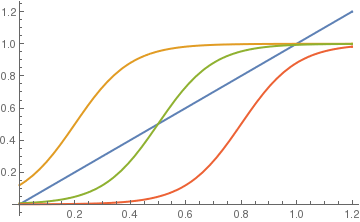Graphs of sigmoidal function S(z) for different values of θ along with y=x.

The equilibrium solutions of this slope functions are constant values of x, where $$x = S(x+E-\theta ) .$$ The figure above shows graphs of y = x and the response function $$y = S(x+E-\theta )$$ for values θ = 0.4, 0.7, and 1.0 with a = 10 and E=0.2. It is seen that for small θ there will be one equilibrium solution near x = 1 and for large θ there will be one stationary solution near x = 0. This seems reasonable because a high threshold means it takes a lot of input to produce very much activity. For θ in a middle range, however, there can be three equilibrium solutions. WE want to determine the two bifurcation values of θ at which the number of stationary solutions changes from 1 to 3 and from 3 to 1. First, we plot direction field:

neuron[t_, a_, e_, k_] = 1/(1 + Exp[-a*(t + e - k)])
StreamPlot[{1, neuron[x, 10, 0.2, 0.7] - x}, {t, 0, 2}, {x, -0.2, 1.2},
StreamStyle -> Yellow, FrameStyle -> LightGray, Background -> Black, FrameLabel -> {t, x}]

1. Abelson, H., The bifurcation interpreter: a step towards the automatic analysis of dynamic systems, Computers & Mathematics with Applications, 1990, Vol. 20, Issue 8, pp. 13--35.